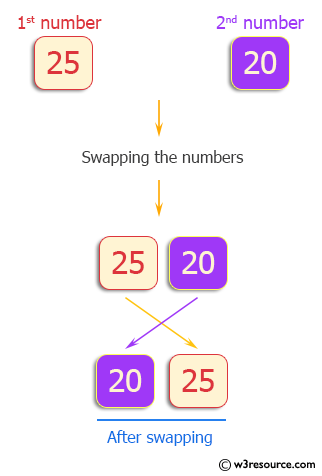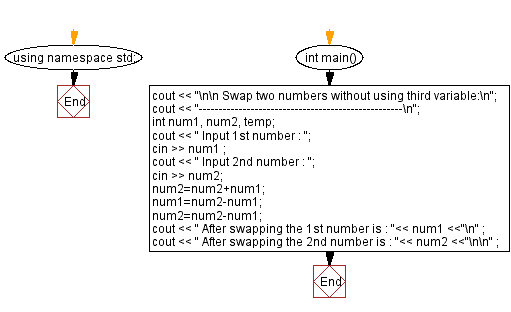﻿ C++ : Swap two variables not using third variable

# C++ Exercises: Swap the values of two variables not using third variable

## C++ Basic: Exercise-48 with Solution

Write a C++ program that swaps two variables without using a third variable.

Pictorial Presentation:Sample Solution:

C++ Code :

``````#include <iostream>
using namespace std;

int main()
{
cout << "\n\n Swap two numbers without using third variable:\n";
cout << "---------------------------------------------------\n";
int num1, num2, temp;
cout << " Input 1st number : ";
cin >> num1 ;
cout << " Input 2nd number : ";
cin >> num2;
num2=num2+num1;
num1=num2-num1;
num2=num2-num1;
cout << " After swapping the 1st number is : "<< num1 <<"\n" ;
cout << " After swapping the 2nd number is : "<< num2 <<"\n\n" ;
}
``````

Sample Output:

```Swap two numbers without using third variable:
---------------------------------------------------
Input 1st number : 25
Input 2nd number : 20
After swapping the 1st number is : 20
After swapping the 2nd number is : 25
```

Flowchart:C++ Code Editor:

What is the difficulty level of this exercise?

﻿

## C++ Programming: Tips of the Day

Why is there no std::stou?

The most pat answer would be that the C library has no corresponding "strtou", and the C++11 string functions are all just thinly veiled wrappers around the C library functions: The std::sto* functions mirror strto*, and the std::to_string functions use sprintf.

Ref: https://bit.ly/3wtz2qA

We are closing our Disqus commenting system for some maintenanace issues. You may write to us at reach[at]yahoo[dot]com or visit us at Facebook